# Correlation Analysis

The following article is from The Great Soviet Encyclopedia (1979). It might be outdated or ideologically biased.

## Correlation Analysis

the aggregate of methods, based on the mathematical theory of correlation, for finding the correlation between two random attributes or factors. Correlation analysis of experimental data includes the following fundamental practical methods: (1) the construction of scatter diagrams and the compilation of correlation tables, (2) the calculation of sample correlation coefficients or correlation ratios, and (3) testing of a statistical hypothesis concerning the significance of a relationship. Further investigation consists of establishing the specific form of the relationship between the quantities. The relationship between three or more random attributes or factors is studied by the methods of multi-dimensional correlation analysis (computation of partial and multiple correlation coefficients and correlation ratios).

Scatter diagrams and correlation tables are auxiliary methods in the analysis of sampled data. A scatter diagram is obtained by plotting the sample points on a coordinate plane. By the nature of the arrangement of the points on the diagram, it is possible to form a preliminary opinion about the form of the relationship of the random quantities (for example, whether, on the average, one quantity increases or decreases with an increase in the other). For numerical analysis, the results are usually grouped and presented in the form of a correlation table. Each location in the correlation table (see) contains the frequencies nij of those (x, y) pairs whose components fall within the corresponding group intervals in each variable.

Assuming the lengths of the group intervals (in each of the variables) are equal, we choose the centers xi (and respectively yj) of the intervals and the numbers nij as the bases for calculation.

The correlation coefficient and the correlation ratio provide more precise information on the nature and the measure of the relationship than does the scatter diagram. The sample correlation coefficient is defined by the formula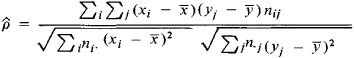where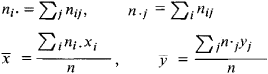For a large number of independent observations obeying the same distribution law and for a proper choice of group intervals, the coefficient ρ̂ is close to the true correlation coefficient ρ. Therefore, the use of ρ̂ as a measure of relationship has a sharply defined meaning for those distributions for which ρ may serve as a natural measure of relationship (that is, for normal or almost normal distributions). In all other cases, it is recommended to use the correlation ratio η, whose interpretation does not depend on the form of the relationship being studied, as a characteristic of the strength of the relationship. The sample value ηY/X is computed from the data in the correlation table: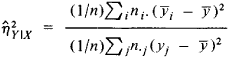where the numerator characterizes the scatter of the conditional mean values yi = Σjnijj/ni near the unconditional mean ȳ (the sample value η̂2x/y is analogously defined). The quantity η̂2x/y — ρ2 is used as a measure of the deviation of the relationship from linearity, since usually η̂2x/y > ρ2 and η̂2x/y > ρ2 and only in the case of a linear relationship does ρ2 = η̂2x/y Thus, in the analysis of the correlation between the heights and the diameters of northern pines, it has been found that the conditional mean values of the heights of the pines for a given diameter are linked by a nonlinear relationship. The correlation ratio (of height to diameter) in this case equals 0.813, and the coefficient of correlation equals 0.762.

Testing of a hypothesis concerning the significance of a relation is based on a knowledge of the laws of the distribution of sample correlation characteristics. In the case of a normal distribution, the value of the sample correlation coefficient ρ is considered to be significantly different from zero if the inequality

(ρ̂)2 > [1 + (n − 2)/tα2]−1

is fulfilled, where is the critical value of Student’s t-distribution with (n — 2) degrees of freedom, which corresponds to a chosen significance level a. However, if it is known that ρ =£ 0, then it is necessary to use Fisher’s z-transformation (which does not depend on ρ or n):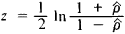It is possible to determine confidence intervals for the true correlation coefficient p from the approximate normality of z.

In the case when the attributes being studied are not quantitative but qualitative, the usual measures of relationship do not apply. However, if one can order the objects being studied with respect to some attribute, that is, assign to them sequential numbers— ranks (two numbers corresponding to the two attributes) —then one may use as a characteristic of relationship, for example, the rank-difference correlation coefficient: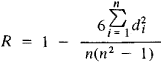where di is the difference between the ranks of the two attributes for each object. According to the degree of deviation of R from zero, it is possible to draw certain conclusions about the degree of relationship between the qualitative attributes. For small samples, the hypothesis of independence of attributes is tested with the aid of special tables, and for n > 10 the fact that the correlation coefficients are approximately normally distributed is used to compute critical values of these coefficients.

A. V. PROKHOROV

References in periodicals archive ?
His topics include psychiatric research, one-variable descriptive statistics, probability and probability distributions, basic elements of statistical inference, experimental data analysis: ANOVA, correlation analysis and regression analysis, survival analysis and validity analysis, multivariate statistical methods, discrimination analysis, reporting the results, and Statistical Package for Social Sciences (SPSS).
Bivariate correlation analysis was performed to analyses the relationship between these significant variables and the occurrence of a PLT count <125x[10.sup.9]/L at POM 3.
Linear correlation analysis and multiple linear regression analysis were used for correlation analysis.
(NASDAQ: GSUM), the Gridsum Big Data Platform and the Gridsum Prophet: Enterprise AI Engine, is built on a distributed computing framework and performs real-time multi-dimensional correlation analysis of both structured and unstructured data.
Bi-variate Pearson Correlation analysis was accompanied on the Pareto Principle and five levels of leadership to check the initial support for the hypothesized relationship of presented in figure.2 the initial outputs showed that Pareto Principle and Maxwell's levels of leadership (r=.454) was significantly correlated.
Table-IV correlation analysis of ACR with different parameters of the two genders.
The oil/source rock correlation analysis using biomarker data shows that the oils in Prabumulih field is correlated with the oils in source rock of Talang Akar Formation.
For correlation between thiol and disulfide values with climacteric symptoms, the Pearson analysis was used for parameters with normal distribution, while the Spearman correlation analysis was used for those without normal distribution.
Therefore, we carried out a correlation analysis between the temperament of the residents of prefectures and the regional characteristics of prefectures and the data on stray IoT devices investigated by prefecture in Japan.
Earlier researchers carried out the correlation analysis studies to probe the effects of different morphological and physiological traits on grain yield under moisture stress condition (Ahmad et al., 2013b).
Across the seven months from April to October, correlation analysis shows that for every transaction recorded online two and a half were recorded in store.

Site: Follow: Share:
Open / Close